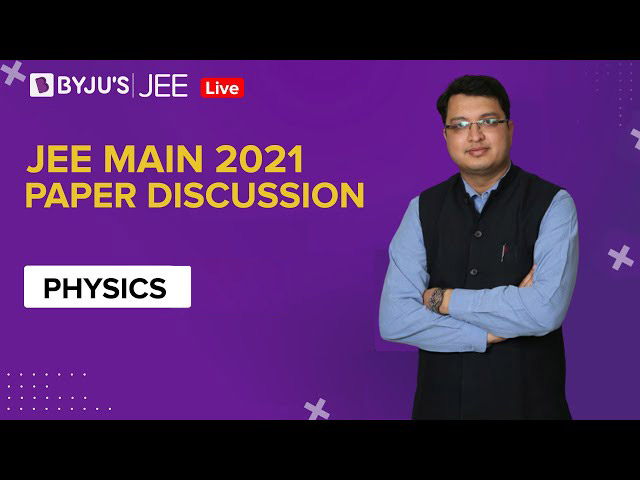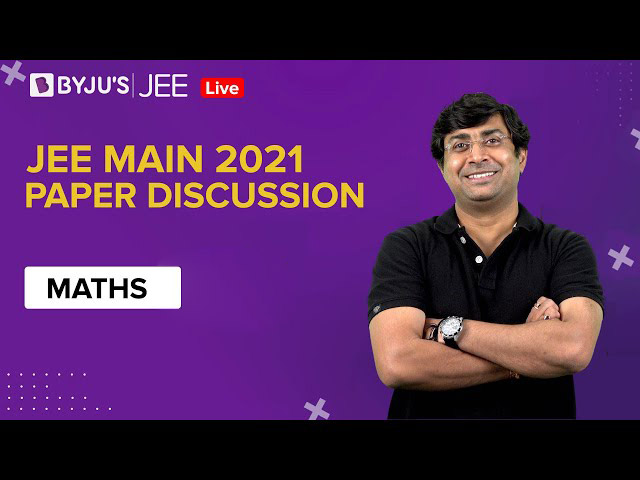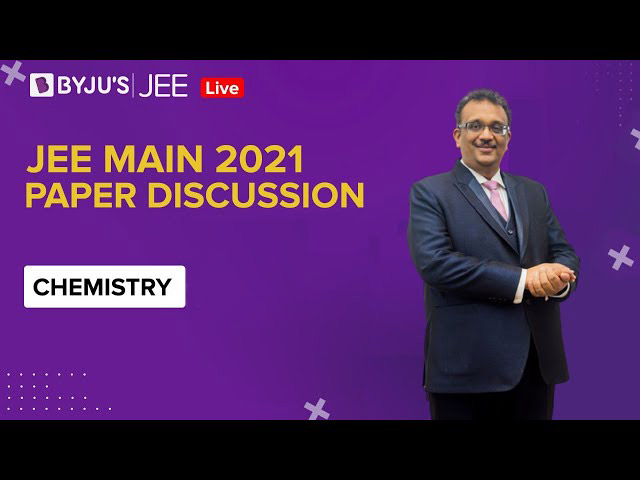Win up to 100% scholarship on Aakash BYJU'S JEE/NEET courses with ABNAT Win up to 100% scholarship on Aakash BYJU'S JEE/NEET courses with ABNAT

# JEE Main 2021 February 26 – Shift 1 Physics Question Paper with Solutions

BYJU’S is providing JEE Main Physics shift 1 question paper along with the solutions for the exam conducted by NTA on 26th February 2021. Candidates will find the Physics memory-based questions and these will help them to check the correct answers and analyze their performance. Subject experts have provided a detailed explanation of each question asked in JEE Main 2021 Physics Paper. Find the video below to get familiar with the JEE Main exam pattern, the marking scheme and the type of questions that are asked in the exam.

JEE Main 2021 26th February Shift 1 Physics Question Paper

SECTION A

Question 1: If λ1 and λ2 are the wavelengths of the third member of Lyman and the first member of the Paschen series respectively, then the value of λ1 : λ2 is:

a. 1 : 3
b. 1 : 9
c. 7 : 135
d. 7 : 108

For Lyman series

n1 = 1, n2 = 4

1/λ1 = RZ2(1/n12 – 1/n22)

1/λ1 = RZ2(1/12 – 1/42)

1/λ1 = 15RZ2/16

λ1 = 16/15RZ2

For Paschen series

n1 = 3, n2 = 4

1/λ2= RZ2(1/32 – 1/42)

1/λ2= RZ2(16-9)/9×16

1/λ2 = RZ2 (7/9×16)

λ2 = 9×16/7RZ2

So,

λ12 = (16/15 RZ2)/(9×16)/7 RZ2

= 16×7/15×9×16

= 7/135

= 7 : 135

Question 2: The temperature θ at the junction of two insulating sheets, having thermal resistances R1 and R2 as well as the bottom and top temperatures θ1 and θ2 (as shown in the figure) is given by: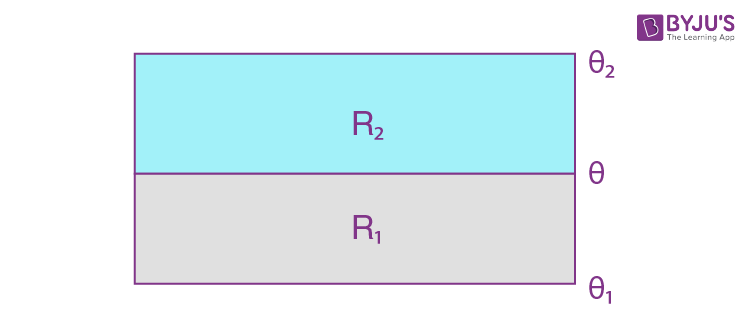a. (θ1R2 + θ2R1)/(R1 + R2)
b. (θ1R2 – θ2R1)/(R2 – R1)
c. (θ2R2 – θ1R1)/(R2 – R1)
d. (θ1R1 + θ2R2)/(R1 + R2)

Temperature at the junction is θ.

So, using the formula,

(T2 – T)/R2 = (T – T1)/R1

2 – θ)/R2 = (θ – θ1)/R1

R12 – θ) = R2(θ – θ1)

R1θ2 – R1θ = R2θ – R2θ1

R1θ + R2θ = R1θ2 + R2θ1

θ = (R1θ2 + R2θ1)/(R1+R2)

Question 3: In Young’s double-slit experiment two slits are separated by 2 mm and the screen is placed one meter away. When light of wavelength 500 nm is used, the fringe separation will be:

a. 0.75 mm
b. 0.50 mm
c. 1 mm
d. 0.25 mm

Fringe width (β) = λD/d

d = 2×10–3 m

λ = 500×10–9 m

D = 1 m

Now,

β = 500×10-9×1/2×10-3

β = (5/2)×10-4

β = 2.5 × 10–4

β = 0.25 mm

Question 4: Given below are two statements: one is labelled as Assertion A and the other is labelled as Reason R.

Assertion A: An electron microscope can achieve better resolving power than an optical microscope.

Reason R: The de Broglie’s wavelength of the electrons emitted from an electron gun is much less than the wavelength of visible light.

In light of the above statements, choose the correct answer from the options given below:

a. A is true but R is false.
b. Both A and R are true but R is NOT the correct explanation of A.
c. Both A and R are true and R is the correct explanation of A.
d. A is false but R is true.

Resolution limit (Δθ) = 1.22λ/d

Resolution power = 1/Resolution limit

λ ↓ Δθ↓

Δθ↓ Power ↑

Question 5: Four identical solid spheres each of mass ‘m’ and radius ‘a’ are placed with their centres on the four corners of a square of side ‘b’. The moment of inertia of the system about one side of square where the axis of rotation is parallel to the plane of the square is:

a. ⅘ ma2
b. (8/5) ma2 + mb2
c. ⅘ ma2 + 2mb2
d. (8/5) ma2 + 2mb2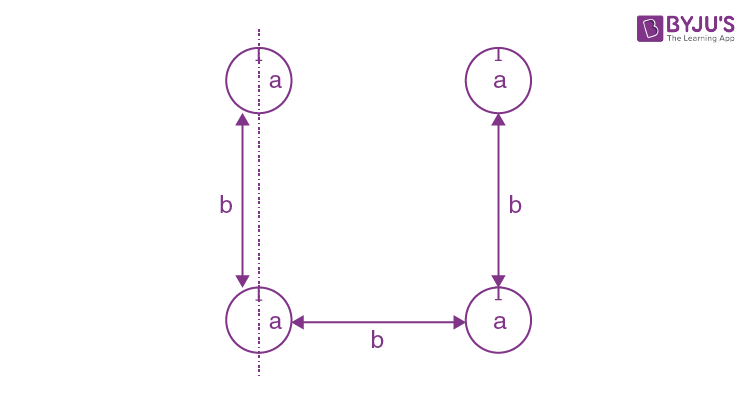I = ⅖ ma2 + ⅖ ma2 + [⅖ ma2+mb2 ]+ [⅖ ma2 + mb2]

I = 4 ×(⅖)ma2 + 2mb2

= 8/5 ma2 + 2mb2

Question 6: The normal density of a material is ρ and its bulk modulus of elasticity is K. The magnitude of increase in density of material when a pressure P is applied uniformly on all sides, will be:

a. ρK/P
b. K/ρP
c. PK/ρ
d. ρP/K

Bulk modulus K = -P/(ΔV/V)

We know, ρ = M/V

So, -Δρ/ρ = ΔV/V

K = -ΔP/(-Δρ/ρ) = ρΔP/Δρ

Δρ = ρΔP/K

Δρ = ρP/K

Question 7: LED is constructed from Ga-As-P semiconducting material. The energy gap of this LED is 1.9 eV. Calculate the wavelength of light emitted and its colour.

[h = 6.63×10–34 Js and c = 3×108 ms–1]

a. 654 nm and red colour
b. 1046 nm and blue colour
c. 1046 nm and red colour
d. 654 nm and orange colour

We know that E = hc/λ

= hc/E ⇒λ( in nm)=1240(in  eV)/E (in eV)

λ = 1240/1.9

= 652.63 nm ≈ 654 nm

The wavelength of red light is 620 nm to 750 nm

Question 8: Five equal resistances are connected in a network as shown in the figure. The net resistance between points A and B is: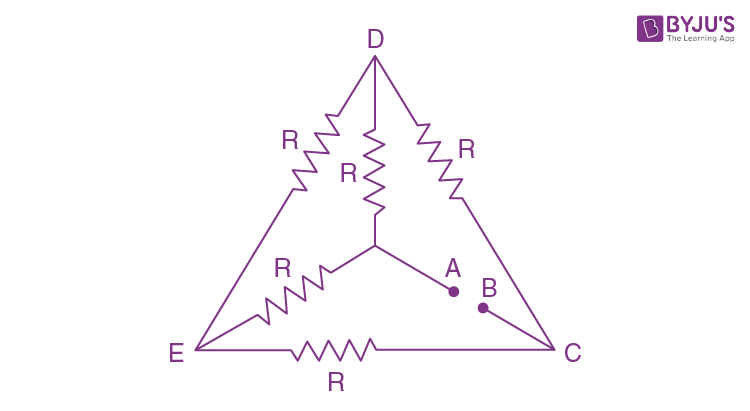a. 3R/2
b. R/2
c. R
d. 2R

It is a balanced Wheatstone bridge.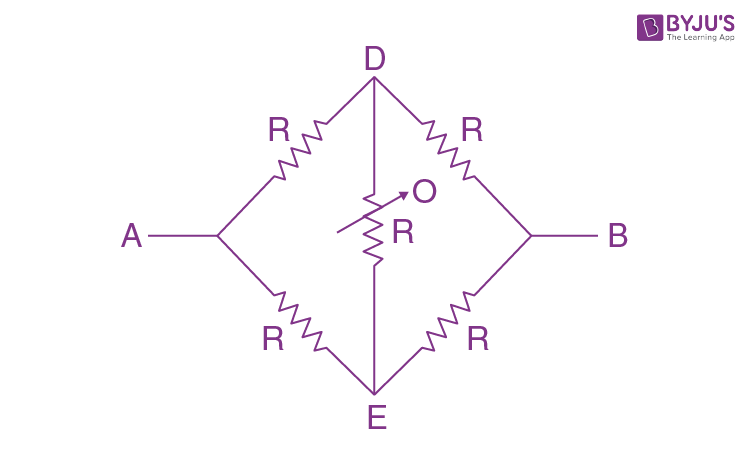So, we know that

R1R4 = R2R3

R1/R2 = R3/R4

If this condition satisfies then RDE become redundant and here it satisfies the equations. Thus, RDE is redundant.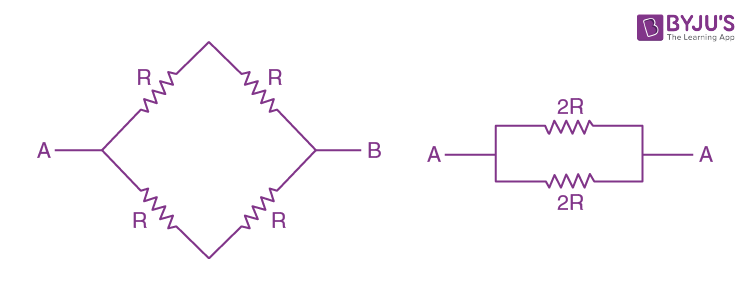Req = 2R×2R/(2R+2R)

= 4R2/4R

= R

Question 9: Find the gravitational force of attraction between the ring and sphere as shown in the diagram, where the plane of the ring is perpendicular to the line joining the centres. If √8R is the distance between the centres of a ring (of mass ‘m’) and a sphere (of mass ‘M’) where both have equal radius ‘R’.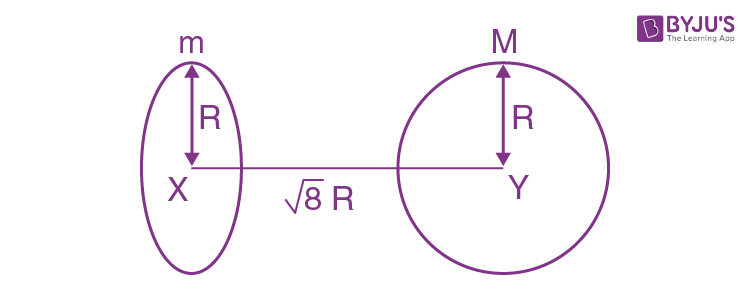a. (√8/9) GmM/R
b. (√8/27) GmM/R2
c. (2√2/3) GMm/R2
d. (1/3√8) GMm/R2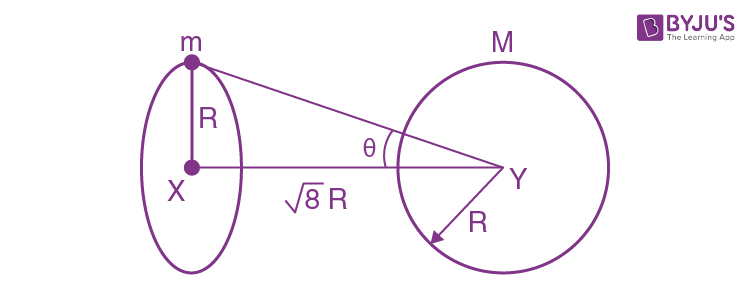We know that,

F = ME = M(Gm√8R/(R2+(√8R)2)3/2

F = GMm8√R/(9R2)3/2

⇒ √8GmM/(9R2)3/2

= √8GmM/27R2

F = √8GMm/27R2

Question 10: Assume that a tunnel is dug along a chord of the earth, at a perpendicular distance (R/2) from the earth’s centre, where ‘R’ is the radius of the Earth. The wall of the tunnel is frictionless. If a particle is released in this tunnel, it will execute a simple harmonic motion with a time period:

a. 2π√R/g
b. (1/2π)√(g/R)
c. 2πR/g
d. g/2πR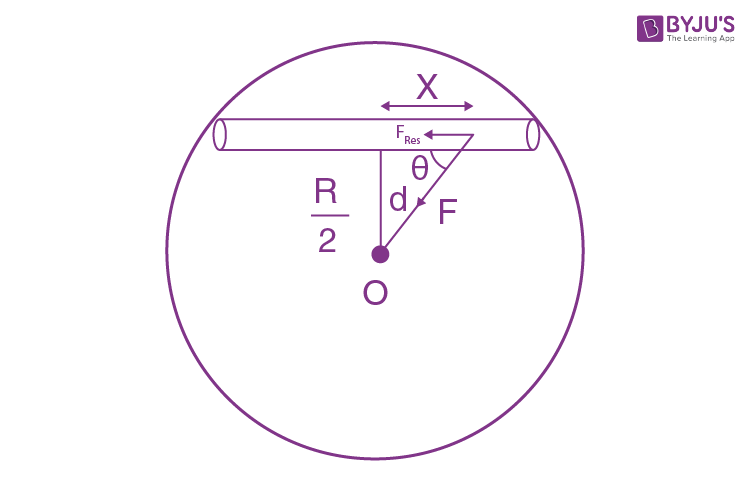cos θ = x/d

If displaced from equilibrium position,

Frestoring = (GMmd/R3)cosθ

Frestoring = (GMmd/R3)x/d = GMmx/R3

a = GMx/R3

We know that the time period is given by,

$$\begin{array}{l}T = 2\pi \sqrt{\left | \frac{x}{a} \right |}\end{array}$$
$$\begin{array}{l}T = 2\pi \sqrt{\frac{\frac{X}{GM_{X}}}{R^{3}}}\end{array}$$

T = 2π√(R/g)

Question 11: Find the electric field E at point P (as shown in the figure) on the perpendicular bisector of a uniformly charged thin wire of length L carrying a charge Q. The distance of the point P from the centre of the rod is a = (√3/2)L.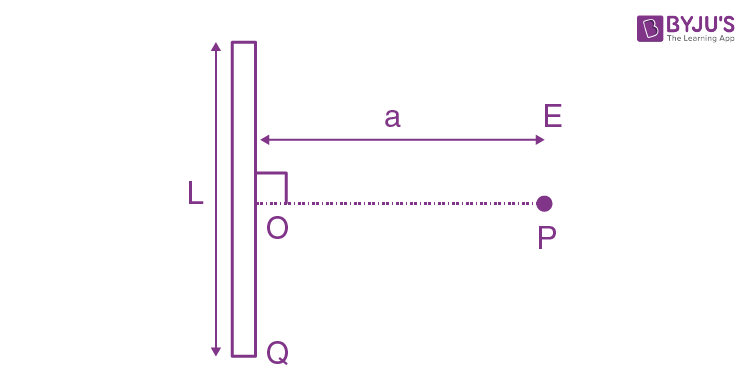a. Q/2√3πε0L2
b. √3Q/4πε0L2
c. Q/3πε0L2
d. Q/4πε0L2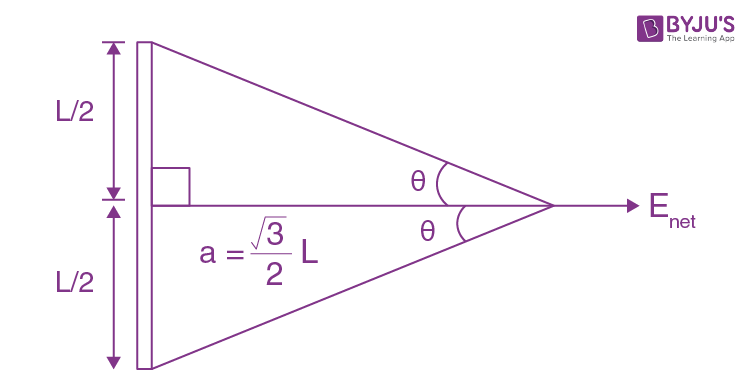tanθ = L/2/(√3/2)L = 1/√3

⇒ θ = 300

Enet = (Kλ/(√3L/2))(sin 300 + sin 300)

⇒ (2KQ/√3L2)(½ + ½ )

Enet = (1/4πε0) (2Q/√3L2)

Enet = Q/2√3πε0L2

Question 12: Given below are two statements: one is labelled as Assertion A and the other is labelled as Reason R.

Assertion A: Body ‘P’ having mass M moving with speed ‘u’ has head-on collision elastically with another body ‘Q’ having mass ‘m’ initially at rest. If m<<M, body ‘Q’ will have a maximum speed equal to ‘2u’ after collision.

Reason R: During elastic collision, the momentum and kinetic energy are both conserved.

In the light of the above statements, choose the most appropriate answer from the options given below:

a. A is correct but R is not correct.
b. Both A and R are correct but R is NOT the correct explanation of A.
c. A is not correct but R is correct.
d. Both A and R are correct and R is the correct explanation of A.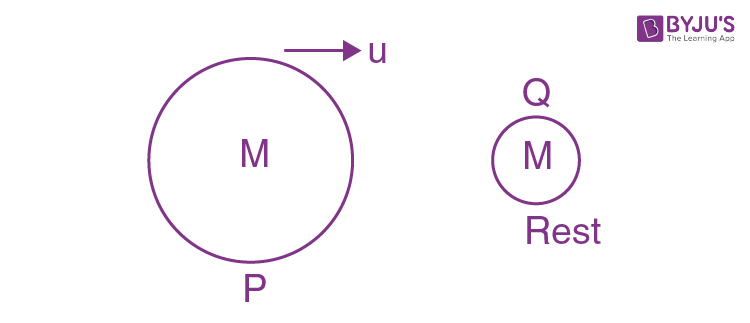m << M

e = (v2 – v1)/(u1-u2)

For elastic collision, e = 1 and

1 = (v2 – u)/(u-0)

u = v2 – u

v2 = 2u

In elastic collision kinetic energy & momentum are conserved.

Question 13: A short straight object of height 100 cm lies before the central axis of a spherical mirror whose focal length has absolute value |f| = 40 cm. The image of the object produced by the mirror is of height 25 cm and has the same orientation as the object. One may conclude from the information:

a. Image is real, same side of concave mirror.
b. Image is virtual, opposite side of convex mirror.
c. Image is virtual, opposite side of concave mirror.
d. Image is real, same side of convex mirror.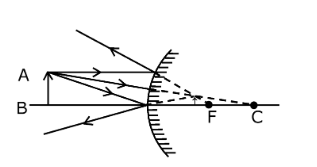Image is upright with respect to the object, this is only possible in the case of a virtual image. The height of image is less than the height of the object, this is possible only in convex mirrors.

Question 14: A particle is moving with uniform speed along the circumference of a circle of radius R under the action of a central fictitious force F which is inversely proportional to R3. Its time period of revolution will be given by:

a. T ∝ R5/2
b. T ∝ R2
c. T ∝R4/3
d. T ∝ R3/2

F∝1/R3

F = K/R3

mv2/R = K/R3

m(ωR)2 = K/R2

2R2 = K/R2

ω2 = (K/m)(1/R4)

(2π/T)2 ∝ 1/R4

2/T2 ∝ 1/R4

T∝R2

Question 15: A large number of water drops, each of radius r, combine to have a drop of radius R. If the surface tension is T and mechanical equivalent of heat is J, the rise in heat energy per unit volume will be:

a. 2T/rJ
b. 3T/rJ
c. (2T/J)(1/r – 1/R)
d. (3T/J)(1/r -1/R)

R is the radius of bigger drop.

r is the radius of smaller drops.

Water drops are combined to make bigger drop.

So,

Volume of n drops = volume of bigger drop

(3T/J)(1/r – 1/R)

⇒ n = (R/r)3

∆U = T(Change in surface area)

∆U = T(n4πr2 – 4πR2)

∆U = 4πT(R3/r) – R2

⇒ H = ∆U/J = 4πT(R3/r – R2)/J

Now, H/V = 4πT(R3/r – R2)/J×(4/3) πR3

= (3T/J)[1/r – 1/R]

Question 16: A planet revolving in elliptical orbit has:

A. a constant velocity of revolution.

B. has the least velocity when it is nearest to the sun.

C. its areal velocity is directly proportional to its velocity.

D. areal velocity is inversely proportional to its velocity.

E. to follow a trajectory such that the areal velocity is constant.

Choose the correct answer from the options given below:

a. A only
b. E only
c. D only
d. C only

From the law of conservation of angular momentum, Li = Lf

mv1r1 = mv2r2 = constant …………..(1)

As the orbit of the planet is elliptical, the direction of velocity will change at each instant. So, velocity will always change.

From (1), speed is minimum when distance from the sun is maximum.

Also,

$$\begin{array}{l}\frac{d\vec{A}}{dt}=\frac{\vec{L}}{2m}\end{array}$$

= constant, thus it does not depend on velocity.

Question 17: An alternating current is given by the equation i = i1sinωt + i2cosωt. The rms current will be:

a. ½ (i12 + i22)1/2
b. (1/√2) (i12 + i22)1/2
c. (1/√2) (i1 + i2)2
d. (1/√2) (i1 + i2)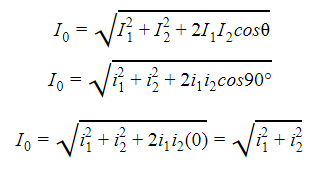We know that,

Irms = I0/√2

So, Irms = √(i12 + i22)/√2

Question 18: Consider the combination of 2 capacitors C1 and C2, with C2>C1, when connected in parallel, the equivalent capacitance is 15/4 times the equivalent capacitance of the same connected in series. Calculate the ratio of capacitors, C2/C1.

a.15/11
b.29/15
c.15/4
d. 111/80

C1 + C2 = (15/4)C1C2/(C1 + C2)

4 (C1 + C2)2 = 15C1C2

4C12 + 4C22 – 7C1C2 = 0

4 + 4 (C2/C1)2– 7C2/C1 = 0

4(C2/C1)2– 7C2/C1 + 4 = 0

4x2 – 7x + 4 = 0 [x = C2/C1]

Here, the determinant is negative, thus, x is imaginary.

C2/C1 = imaginary

Question 19: If two similar springs each of spring constant K1 are joined in series, the new spring constant and time period would be changed by a factor:

a. ½, √2
b. ¼, 2√2
c. ½, 2√2
d. ¼ , √2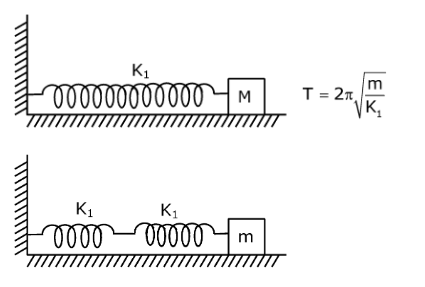Keq = K1 ×K1/(K1+K1) = K12/2K1

Keq = K1/2

T’ = 2π√(m/Keq )

= 2π√(m/(K1/2))

= √2T

Question 20: In a typical combustion engine the work done by a gas molecule is given by

$$\begin{array}{l}W= \alpha ^{2}\beta e^{\frac{-\beta x^{2}}{kT}}\end{array}$$
, where x is the displacement, k is the Boltzmann constant and T is the temperature. If α and β are constants, dimensions of α will be:

a. [M0 L T0]
b. [M2 L T–2]
c. [M L T–2]
d. [M L T-1]

βx2/kT is dimensionless

So,

[kT] = [βx2]

⇒[M1L2T–2] = [β][L2] [β] = [M1L2T-2]/[L2] = [M1T–2]

Now, [W] = [α2β] [M1L2T–2] = [α2][M1T–2] [α2] = [L2] [α ]=[ L ] [α] =[ M0L1T0]

Section – B

Question 1: The mass per unit length of a uniform wire is 0.135 g/cm. A transverse wave of the form y = –0.21sin(x+30t) is produced in it, where x is in meter and t is in second. Then, the expected value of tension in the wire is x×10–2 N. Value of x is __________. (Round-off to the nearest integer)

y = -0.21 sin(x + 30t)

Mass per unit length (μ) = 0.135 g/cm = 0.135 × 10-3/10-2 kg/m

v = ω/k = 30/1 = 30 m/s

v = √(T/μ)

T = v2 × μ

T = (30)2 × 0.135 ×10–1

T = 900 × 0.135 ×10–1

T = 12.15 N

T = 1215 × 10–2 N

x = 1215

Question 2: A radiation is emitted by a 1000 W bulb, and it generates an electric field and magnetic field at P, placed at a distance of 2 m. The efficiency of the bulb is 1.25%. The value of the peak electric field at P is x × 10–1 V/m. Value of x is __________. (Rounded-off to the nearest integer)

[Take ε0 = 8.85×10–12 C2 N–1 m–2, c = 3×108 ms–1]

Intensity of electromagnetic wave is,

I = ½ cε0 E02 = P/4πr2

½ ×4πε0×c×E02 = P/r2

½ 3×108 × E02/9×109 = (1000×1.25/(2)2 )×1/100

E02 = 60×1000×1.25/4×100 = 125×3/2

E02= 375/2 = 187.5

E0= 13.69

E0 ≈ 137×10-1 V/m

x = 137

Question 3: The circuit contains two diodes each with a forward resistance of 50 Ω and with infinite reverse resistance. If the battery voltage is 6 V, the current through the 120 Ω resistance is _________ mA.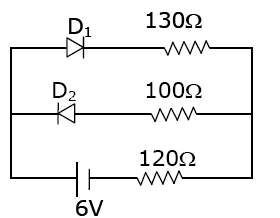D2 is reverse biased so current does not flow through D2.

D1 is forward biased.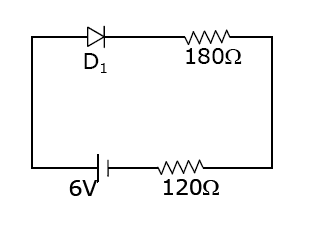I = 6/300

I = 0.02  A

= 20 mA

Question 4: As shown in the figure, a block of mass √3 kg is kept on a horizontal rough surface of coefficient of friction 1/3√3. The critical force to be applied on the vertical surface as shown at an angle 600 with horizontal such that it does not move will be 3x. The value of x will be _______ (upto two decimal places).

[g =10 m/s2; sin 600 = √3/2; cos 600 = ½]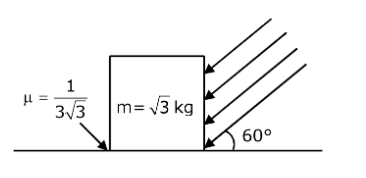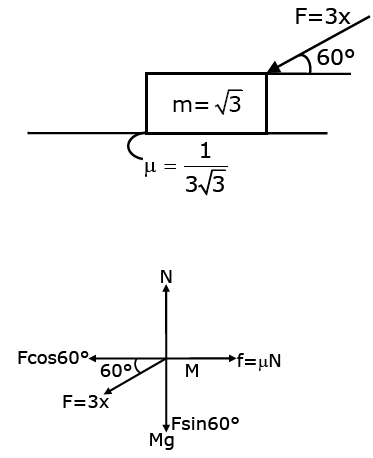N = mg + Fsin600

N = √3g + F√3/2

For no slipping,

F cos 600 = Friction force

F/2 = μN = (1/3√3)(√3g + F√3/2)

F/2 = g/3 + F/6

F/2 – F/6 = g/3

(6F- 2F)/12 = g/3

4F = 4g

F = 10

F = 3x

x = F/3 = 10/3 = 3.33

x = 3.33

Question 5: In a series LCR resonant circuit, the quality factor is measured as 100. If the inductance is increased by two fold and resistance is decreased by two fold, then the quality factor after this change will be __________. (up to two decimal places).

Quality factor = XL/R = ωL/R

Q = (1/√(LC)) (L/R)

Q = (1/√C)(√L/R

Q’ = √(2L)/(R/2)√C = 2√2 Q

Q’ = 282.84

Question 6: In an electrical circuit, a battery is connected to pass 20 C of charge through it in a certain given time. The potential difference between two plates of the battery is maintained at 15 V. The work done by the battery is __________ J.

Charge flown (Q) = 20 C

Potential difference (V) = 15 V

Work done (w) = Q.V

⇒ 20 × 15 = 300 J

Question 7: A container is divided into two chambers by a partition. The volume of the first chamber is 4.5 litre and the second chamber is 5.5 litre. The first chamber contains 3.0 mole of gas at pressure 2.0 atm and the second chamber contain 4.0 mole of identical gas at pressure 3.0 atm. After the partition is removed and the mixture attains equilibrium, then, the common equilibrium pressure existing in the mixture is x×10–1 atm. Value of x is _______.

By energy Conservation

(f/2) n1RT1 +(f/2) n2RT2 = (f/2)(n1 + n2)RT

Using PV = nRT

P1V1 + P2V2 = P(V1 + V2)

P = (P1V1 + P2V2 )/(V1 + V2)

P = (2×4.5 + 3×5.5)/(4.5 + 5.5)

P = (9 + 16.5)/10 = 25.5/10

≈ 26 × 10–1 atm

Question 8: A boy pushes a box of mass 2 kg with a force

$$\begin{array}{l}\vec{F}=(20\hat{i}+10\hat{j})N\end{array}$$
on a frictionless surface. If the box was initially at rest, then __________m is displacement along the x-axis after 10 s.

$$\begin{array}{l}\vec{F}=(20\hat{i}+10\hat{j})N\end{array}$$

Fx = 20 N

Fy = 10 N

ax = Fx/M = 20/2 = 10 m/s2

Displacement on x-axis is

Sx = uxt + ½ axt2

Sx = 0 × 10 + ½ × 10 ×(10)2

= 500  m

Question 9: The maximum and minimum amplitude of an amplitude-modulated wave is 16 V and 8 V respectively. The modulation index for this amplitude modulated wave is x ×10–2. The value of x is ________.

Am = (Amax– Amin)/2

AC = (Amax+ Amin)/2

Modulation index (mi) =Am/AC = (Amax – Amin)/2/(Amax + Amin)/2

= (Amax– Amin)/(Amax+ Amin)

mi = (16-8)/(16+8)

= 8/24

= ⅓

= 0.33

mi = 33 × 10–2

x = 33

Question 10: A person standing on a spring balance inside a stationary lift measures 60 kg. The weight of that person if the lift descends with uniform downward acceleration of 1.8 m/s2 will be ___________ N. [g =10m/s2]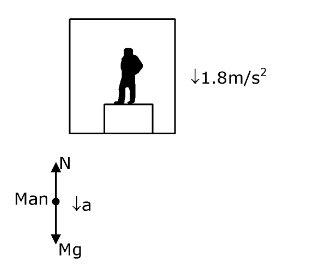Mg – N = Ma

N = M(g–a)

N = 60(10–1.8)

N = 60 × 8.2 = 492 N